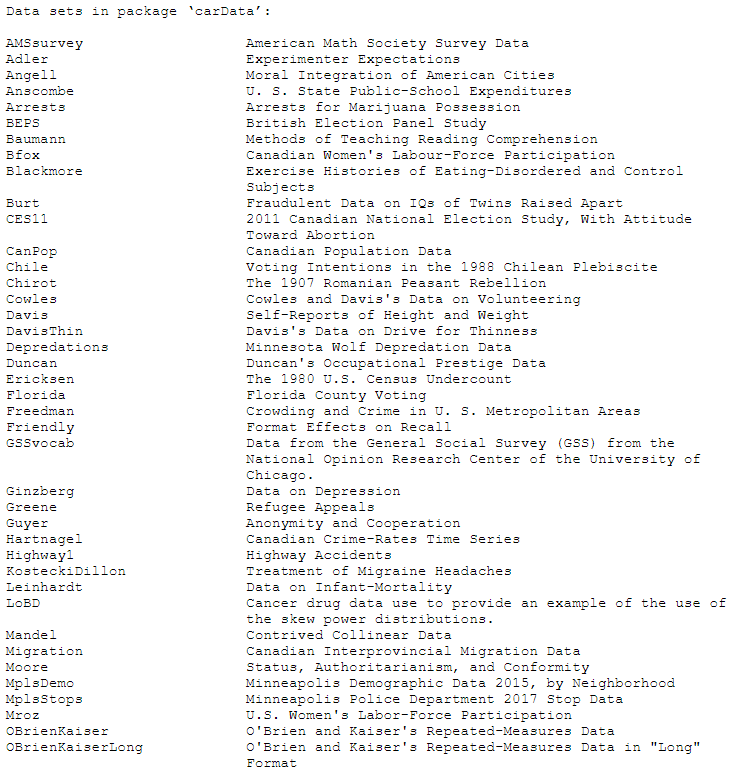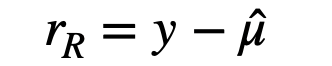1598811480

# Modelling Multiple Linear Regression Using R (research-oriented modelling

### Article Outline

• Dataset description
• Exploratory data analysis
• A simple linear regression model fitting
• Model interpretation
• MLR regression model fitting and interpretation
• Hypothesis testing
• Stepwise regression

### Aim

The aim of this article to illustrate how to fit a multiple linear regression model in the R statistical programming language and interpret the coefficients. Here, we are going to use the Salary dataset for demonstration.

### Dataset Description

The 2008–09 nine-month academic salary for Assistant Professors, Associate Professors and Professors in a college in the U.S. The data were collected as part of the on-going effort of the college’s administration to monitor salary differences between male and female faculty members .

The data frame includes 397 observations and 6 variables.

rank (I1): a factor with levels AssocProf, AsstProf, Prof

discipline (I2): a factor with levels A (“theoretical” departments) or B (“applied” departments).

yrs.since.phd (I3): years since PhD.

yrs.service (I4): years of service.

sex (I5): a factor with levels Female and Male

salary (D): nine-month salary, in dollars.

Where** I: **Independent variable; D: Dependent/Outcome variable

``````## use install.packages( ) function for installation

library(carData)    ## Salary dataset
library(broom)      ## tidy model output
``````

Print dataset details

Let’s print different inbuilt datasets offered by the carData package.

``````data(package = "carData")
``````#machine-learning #coefficient #stepwise #interpretation #regression

## Buddha Community1603170000

## Diagnose the Generalized Linear Models

Generalized Linear Model (GLM) is popular because it can deal with a wide range of data with different response variable types (such as binomial_, Poisson, or _multinomial).Comparing to the non-linear models, such as the neural networks or tree-based models, the linear models may not be that powerful in terms of prediction. But the easiness in interpretation makes it still attractive, especially when we need to understand how each of the predictors is influencing the outcome.The shortcomings of GLM are as obvious as its advantages. The linear relationship may not always hold and it is really sensitive to outliers. Therefore, it’s not wise to fit a GLM without diagnosing.In this post, I am going to briefly talk about how to diagnose a generalized linear model. The implementation will be shown in R codes.There are mainly two types of diagnostic methods. One is outliers detection, and the other one is model assumptions checking.

### Residuals

Before diving into the diagnoses, we need to be familiar with several types of residuals because we will use them throughout the post. In the Gaussian linear model, the concept of residual is very straight forward which basically describes the difference between the predicted value (by the fitted model) and the data.Response residuals

In the GLM, it is called “response” residuals, which is just a notation to be differentiated from other types of residuals.The variance of the response is no more constant in GLM, which leads us to make some modifications to the residuals.If we rescale the response residual by the standard error of the estimates, it becomes the Pearson residual.

#data-science #linear-models #model #regression #r1598811480

## Modelling Multiple Linear Regression Using R (research-oriented modelling

### Article Outline

• Dataset description
• Exploratory data analysis
• A simple linear regression model fitting
• Model interpretation
• MLR regression model fitting and interpretation
• Hypothesis testing
• Stepwise regression

### Aim

The aim of this article to illustrate how to fit a multiple linear regression model in the R statistical programming language and interpret the coefficients. Here, we are going to use the Salary dataset for demonstration.

### Dataset Description

The 2008–09 nine-month academic salary for Assistant Professors, Associate Professors and Professors in a college in the U.S. The data were collected as part of the on-going effort of the college’s administration to monitor salary differences between male and female faculty members .

The data frame includes 397 observations and 6 variables.

rank (I1): a factor with levels AssocProf, AsstProf, Prof

discipline (I2): a factor with levels A (“theoretical” departments) or B (“applied” departments).

yrs.since.phd (I3): years since PhD.

yrs.service (I4): years of service.

sex (I5): a factor with levels Female and Male

salary (D): nine-month salary, in dollars.

Where** I: **Independent variable; D: Dependent/Outcome variable

``````## use install.packages( ) function for installation

library(carData)    ## Salary dataset
library(broom)      ## tidy model output
``````

Print dataset details

Let’s print different inbuilt datasets offered by the carData package.

``````data(package = "carData")
``````#machine-learning #coefficient #stepwise #interpretation #regression1594271340

## A Deep Dive into Linear Regression

Let’s begin our journey with the truth — machines never learn. What a typical machine learning algorithm does is find a mathematical equation that, when applied to a given set of training data, produces a prediction that is very close to the actual output.

Why is this not learning? Because if you change the training data or environment even slightly, the algorithm will go haywire! Not how learning works in humans. If you learned to play a video game by looking straight at the screen, you would still be a good player if the screen is slightly tilted by someone, which would not be the case in ML algorithms.

However, most of the algorithms are so complex and intimidating that it gives our mere human intelligence the feel of actual learning, effectively hiding the underlying math within. There goes a dictum that if you can implement the algorithm, you know the algorithm. This saying is lost in the dense jungle of libraries and inbuilt modules which programming languages provide, reducing us to regular programmers calling an API and strengthening further this notion of a black box. Our quest will be to unravel the mysteries of this so-called ‘black box’ which magically produces accurate predictions, detects objects, diagnoses diseases and claims to surpass human intelligence one day.

We will start with one of the not-so-complex and easy to visualize algorithm in the ML paradigm — Linear Regression. The article is divided into the following sections:

1. Need for Linear Regression

2. Visualizing Linear Regression

3. Deriving the formula for weight matrix W

4. Using the formula and performing linear regression on a real world data set

Note: Knowledge on Linear Algebra, a little bit of Calculus and Matrices are a prerequisite to understanding this article

Also, a basic understanding of python, NumPy, and Matplotlib are a must.

## 1) Need for Linear regression

Regression means predicting a real valued number from a given set of input variables. Eg. Predicting temperature based on month of the year, humidity, altitude above sea level, etc. Linear Regression would therefore mean predicting a real valued number that follows a linear trend. Linear regression is the first line of attack to discover correlations in our data.

Now, the first thing that comes to our mind when we hear the word linear is, a line.

Yes! In linear regression, we try to fit a line that best generalizes all the data points in the data set. By generalizing, we mean we try to fit a line that passes very close to all the data points.

But how do we ensure that this happens? To understand this, let’s visualize a 1-D Linear Regression. This is also called as Simple Linear Regression

#calculus #machine-learning #linear-regression-math #linear-regression #linear-regression-python #python1595201363

## Php how to delete multiple rows through checkbox using ajax in laravel

First thing, we will need a table and i am creating products table for this example. So run the following query to create table.

``````CREATE TABLE `products` (
`id` int(10) unsigned NOT NULL AUTO_INCREMENT,
`name` varchar(255) COLLATE utf8mb4_unicode_ci NOT NULL,
`description` varchar(255) COLLATE utf8mb4_unicode_ci DEFAULT NULL,
`created_at` timestamp NULL DEFAULT CURRENT_TIMESTAMP,
`updated_at` datetime DEFAULT NULL,
PRIMARY KEY (`id`)
) ENGINE=InnoDB AUTO_INCREMENT=7 DEFAULT CHARSET=utf8mb4 COLLATE=utf8mb4_unicode_ci
``````

Next, we will need to insert some dummy records in this table that will be deleted.

``````INSERT INTO `products` (`name`, `description`) VALUES

('Test product 1', 'Product description example1'),

('Test product 2', 'Product description example2'),

('Test product 3', 'Product description example3'),

('Test product 4', 'Product description example4'),

('Test product 5', 'Product description example5');
``````

Now we are redy to create a model corresponding to this products table. Here we will create Product model. So let’s create a model file Product.php file under app directory and put the code below.

``````<?php

namespace App;

use Illuminate\Database\Eloquent\Model;

class Product extends Model
{
protected \$fillable = [
'name','description'
];
}
``````

## Step 2: Create Route

Now, in this second step we will create some routes to handle the request for this example. So opeen routes/web.php file and copy the routes as given below.

routes/web.php

``````Route::get('product', 'ProductController@index');
Route::delete('product/{id}', ['as'=>'product.destroy','uses'=>'ProductController@destroy']);
Route::delete('delete-multiple-product', ['as'=>'product.multiple-delete','uses'=>'ProductController@deleteMultiple']);
``````

#laravel #delete multiple rows in laravel using ajax #laravel ajax delete #laravel ajax multiple checkbox delete #laravel delete multiple rows #laravel delete records using ajax #laravel multiple checkbox delete rows #laravel multiple delete1598352300

## Regression: Linear Regression

Machine learning algorithms are not your regular algorithms that we may be used to because they are often described by a combination of some complex statistics and mathematics. Since it is very important to understand the background of any algorithm you want to implement, this could pose a challenge to people with a non-mathematical background as the maths can sap your motivation by slowing you down.In this article, we would be discussing linear and logistic regression and some regression techniques assuming we all have heard or even learnt about the Linear model in Mathematics class at high school. Hopefully, at the end of the article, the concept would be clearer.

**Regression Analysis **is a statistical process for estimating the relationships between the dependent variables (say Y) and one or more independent variables or predictors (X). It explains the changes in the dependent variables with respect to changes in select predictors. Some major uses for regression analysis are in determining the strength of predictors, forecasting an effect, and trend forecasting. It finds the significant relationship between variables and the impact of predictors on dependent variables. In regression, we fit a curve/line (regression/best fit line) to the data points, such that the differences between the distances of data points from the curve/line are minimized.

#regression #machine-learning #beginner #logistic-regression #linear-regression #deep learning